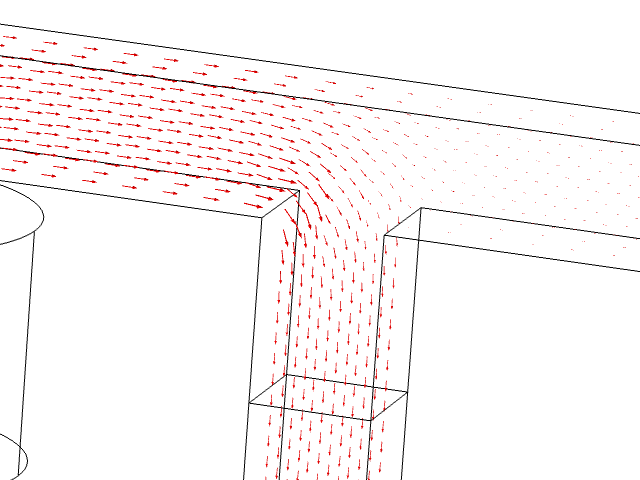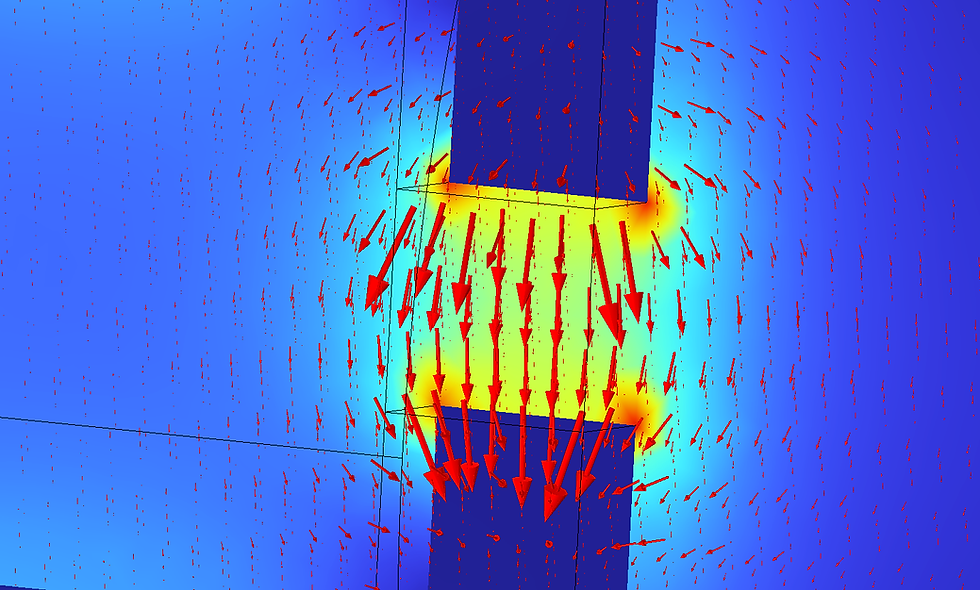top of page

# #041: Lumped Magnetics Circuit

Lumped parameter circuits are used a lot to establish simplified models that represent more complex acoustical, mechanical, or electrical systems. In this blog post we will look at another physics that can be put in a lumped parameter form; magnetics.

We start out with the setup below. A coil is wrapped around a geometry. Three lengths are indicated, l1, l2, and l3, and they are 12 cm, 4 cm, and 12 cm, respectively. Any cross-section is 1 cm by 1 cm.A current is running in the coil, and that generates a magnetic field. Note that we are only looking at static conditions for now, so the low resistance of the coil is of course to be considered in real life to make sure your current source survives. Now, the way to make an equivalent electrical circuit that describes the above situation, is to consider the 'Ohms law' of magnetics:

The magnetomotive force NI is the number of turns in the coil, and I is the current in the coil. On the right hand side is the magnetic flux times the reluctance. The three distinct sections indicated in the figure above show where a single component can be used to describe the section, based on the fact that the flux will split out where they meet. The magnetomotive force will hence act as a voltage in the equivalent circuit, fluxes are currents, and reluctances are resistors. Each reluctance can be calculated as

The l is length of the section, and denominator is permeability (vacuum times relative) times the area of the section. The equivalent lumped circuit then becomesCalculating the flux using a finite element model and the lumped model gives similar results with

 ​ FEM LPM Flux 1 9.3e-6 Wb 8.4e-6 Wb Flux 2 6.7e-6 Wb 6.3e-6 Wb Flux 3 2.0e-6 Wb 2.1e-6 Wb

There are some discrepancies, but we are going for order-of-magnitude correct in this quick setup. There are aspects such as flux leakage to consider, and the sections are not as distinct as the initial drawing indicates, as can be seen from the B-field not 'following' the geometry exactly. So with more care, one might get a better match.With the length of l3 being higher than that of l2, the flux is higher in the l2 section, since the reluctance is lower in this section. Knowing the fluxes, we can calculate the B-fields and H-fields in the different sections. The flux relates to the B-field via

so if the B-field follows the geometry, the flux is simply the B-field times the area, and since flux and area are known, the field can of course be found. The H-field can also be found via

and one also show that the magnetomotive force is also equal to

and using Kirchhoff's laws, one can calculate either flux or field in these closed loops in the geometry.

If we now introduce an airgap, we can see how the fields will vary for a given flux. A 1 cm cut is made in the section opposite of the coil.The section in question can now be thought of having a steel section of length 11, and an air path with a length of 1 cm in series, with each their permeability and reluctance value. The associated equivalent circuit now becomeswith the fourth reluctance coming from the airgap. The total flux coming out of the source will be different than before, because the total reluctance has changed, and the flux distribution through the path in the middle and on the right will also change as the reluctance has increased for the airgap path.

The flux through the middle path is higher than through the path on the right, and this will show in both the simulation and the lumped circuit.But another characteristic is that since there is still flux in the path with the air gap, the B-field will stay fairly constant both through the steel and through the airgap (assuming that there is not too much fringing going on in the airgap, which is not necessarily the case with this gap size). Therefor, the H-field has to be much higher in the airgap, since the permeability is much lower in air compared to steel. And that can again be seen both visualised in a simulation, or calculated in the lumped circuit, but of course the simulation captures many more details about the field distributions.You can now move on to for example harmonic variation of the current in the coil, and/or add permanent magnets, and start to consider more effects such as skin effects. Having just worked on a quite complex structural mechanics/electromagnetics client project, I have had to get into the magnetics theory more, and it has been a very interesting journey.

Lumped parameter modelling is brilliant when possible; it is quick, you can establish analytical transfer functions and sensitivity analysis, and it is fairly easy to see the consequence of changing one or more parameters. Whether you work with acoustics, structural mechanics, magnetics, or any other physics, where you look at statics and/or harmonic variations, I highly recommend getting into lumped modelling.

bottom of page